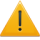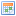# Vector

## Object MethodsThis page needs to be edited as it contains information that is unclear or incorrect. Improvement can be discussed on the talk page. Find more pages that need work here.Details: None given.
 Lua: VectorDescription: Gives a brief explanation of Vector library.Original Author: MerlzothCreated: 1 December 2006

A vector, in the sense used in both Garry's Mod and this documentation, is a quantity represented by three numbers, which express distance from the origin along three directed, perpendicular lines that pass through the origin called the axes. In this setting, vectors can be used to represent position, velocity and rotation in space.

Points can be also represented as vectors from the origin.

## Vector Mathematics

### Reading components of an vector

```
Msg(tostring(vector.x)) //Prints X component of vector
Msg(tostring(vector.y)) //Prints Y component of vector
Msg(tostring(vector.z)) //Prints Z component of vector
```

### Sum

The result of adding two vectors, a and b, is a vector from the tail of a to the head of b.

### Difference

The result of subtracting one vector from another, is a vector from the head of the subtrahend to the head of the minuend.

### Scalar Multiplication

Scalar multiplication is a way of scaling a vector. If the scalar is negative, the vector also undergoes a rotation of 180 degrees.

### Division

Vectors can be divided by scalars, but the result of division by a vector is undefined.

### Dot Product

The product of two vectors returns a scalar, with which the angle between two vectors can be calculated. To get the angle between the two vectors. you take the inverse cosine of the dot product divided by the product of the magnitudes of the two vectors.

### Cross Product

The cross product of two vectors a and b is a pseudovector perpendicular to both a and b.

### Normalization

Not to be confused with the normal of a vector, normalization is the process whereby a vector is made to have unit length, while preserving its original direction. A vector with unit length is said to be a unit vector.

## Code

### Assignment

Example code:

```
local vector = Vector( 0, 0, 1 )
Msg( tostring( vector ) .. "\n" )
```

### Operators

```
local a = Vector( 0, 0, 1 )
local b = Vector( 1, 1, 0 )
Msg( tostring( a + b ) .. "\n" )
```

#### Subtraction

Main page: Vector._sub

```
local a = Vector( 0, 0, 1 )
local b = Vector( 1, 1, 0 )
Msg( tostring( a - b ) .. "\n" )
```

#### Multiplication

Main page: Vector._mul

```
local vector = Vector( 0, 0, 1 )
Msg( tostring( vector * 10 ) .. "\n" )
```

#### Division

Main page: Vector._div

```
local vector = Vector( 0, 0, 1 )
Msg( tostring( vector / 10 ) .. "\n" )
```

#### Equality

Main page: Vector._eq

```
local a = Vector( 0, 0, 1 )
local b = Vector( 1, 0, 0 )
Msg( tostring( a == b and "Equal" or "Not Equal!" ) .. "\n" )
```

### Methods

#### Angle

Main page: Vector.Angle

#### Cross

Main page: Vector.Cross

Returns the cross product of the vector with the argument. Example code:

```
local a = Vector( 0, 0, 1 )
local b = Vector( 1, 0, 0 )
Msg( tostring( a:Cross( b ) ) .. "\n" )
```

#### Distance

Main page: Vector.Distance

#### Dot

Main page: Vector.Dot

#### DotProduct

Main page: Vector.DotProduct

Returns the dot product of the vector with the argument.

```
local a = Vector( 0, 0, 1 )
local b = Vector( 1, 0, 0 )
Msg( tostring( a:DotProduct( b ) ) .. "\n" )
```

#### GetNormal

Main page: Vector.GetNormal

Returns the normal of the vector.

```
local vector = Vector( 0, 0, 1 )
Msg( tostring( vector:GetNormal() ) .. "\n" )
```

#### GetNormalized

Main page: Vector.GetNormalized

Returns the normalized vector.

```
local vector = Vector( 0, 0, 10 )
Msg( tostring( vector:GetNormalized() ) .. "\n" )
```

#### Length

Main page: Vector.Length

Returns the magnitude of the vector.

```
local vector = Vector( 0, 0, 10 )
Msg( tostring( vector:Length() ) .. "\n" );
```

#### Mul

Main page: Vector.Mul

Performs scalar multiplication on the vector and the argument.

```
local vector = Vector( 0, 0, 1 )
vector:Mul( 10 )
Msg( tostring( vector ) .. "\n" )
```

#### Normalize

Main page: Vector.Normalize

Normalizes the vector.

```
local vector = Vector( 0, 0, 10 )
vector:Normalize()
Msg( tostring( vector:GetNormalized() ) .. "\n" )
```

#### Sub

Main page: Vector.Sub